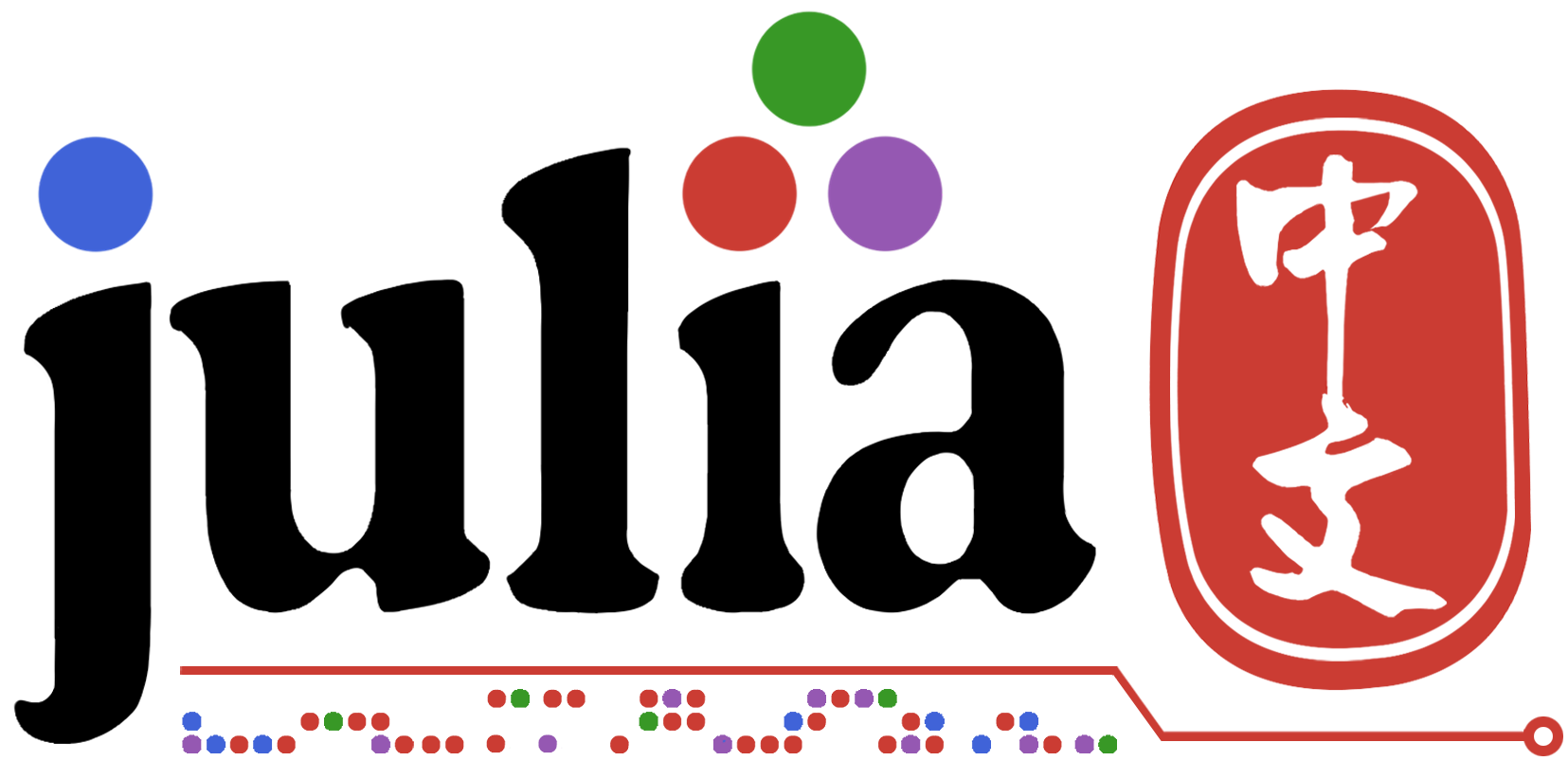# 关于逻辑运算的一点问题

``````1==1 | 1==2
#返回true
1==2 | 1==1
#返回false，这个就是我不能理解的点
(1==2) | (1==1)
#返回true，又正常了
1==2 || 1==1
#返回true，这个不加括号也正常
``````

``````julia> true || error("this never errors")
true

julia> true | error("this could error")
ERROR: this could error
``````
1赞

``````is_valid_arguments(args...) || error("invalid arguments")
``````

``````is_even(x) || (x = x+1)
# equivalent to
x = is_even(x) ? x : x+1
``````